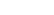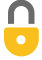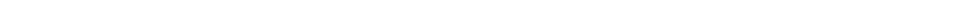Problem 23
Q

# Determine the energy content of 100 g of Karen’s fudge cookies from the following measurements. A 10-g sample of a cookie is allowed to dry before putting it in a bomb calorimeter (page 396). The aluminum bomb has a mass of 0.615 kg and is placed in 2.00 kg of water contained in an aluminum calorimeter cup of mass 0.524 kg. The initial temperature of the system is $15.0 ^\circ \textrm{C}$, and its temperature after ignition is $36.0 ^\circ \textrm{C}$.A
$471 \textrm{ Cal}$In order to watch this solution you need to have a subscription.VIDEO TRANSCRIPT

This is Giancoli Answers with Mr. Dychko. The energy lost by this 10 gram sample of fudge put in the calorimeter is going to equal the energy gained by everything in the calorimeter. There's the aluminum bomb which is the little container that holds the sample, there is the calorimeter cup itself which holds both, the bomb and the water. And then there's the water. So, all 3 things have the same change in temperature because they all start at the same initial temperature and they have the same final temperature. So, we can factor that out, that's ΔTc for calorimeter. And then both, the calorimeter cup and the bomb are both made out of aluminum. So, we can factor the specific heat of aluminum out from both of those terms. And then plus the mass of water times specific heat of water. So, that's 306.0 degrees Celsius, final temperature, minus 15 degrees Celsius, initial temperature, times 900 joules per kilogram Celsius degrees, specific heat of aluminum, times 0.615 kilograms, mass of the calorimeter cup, plus 0.524 kilograms, mass of the bomb, plus 2 kilograms times 4,186 joules per kilogram Celsius degree, that's the mass of water that's inside the calorimeter. And this gives 1.9734 times 10 to the 5 joules which multiplied by 1 Calorie for every 4.186 times 10 to the 3 joules. This gives 47.143 Calories. Now, this is a 10 gram sample, so it's as many Calories per 10 grams. So, to find the Calories in 100 grams, we take 47.143 Calories per 10 grams multiplied by 100 grams. And this gives 471 Calories.

Giancoli Answers, including solutions and videos, is copyright © 2009-2023 Shaun Dychko, Vancouver, BC, Canada. Giancoli Answers is not affiliated with the textbook publisher. Book covers, titles, and author names appear for reference purposes only and are the property of their respective owners. Giancoli Answers is your best source for the 7th and 6th edition Giancoli physics solutions.